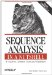cpgplot

 cpgplot

cpgplot plots CpG rich areas.

Here is a sample session with cpgplot:

% cpgplot embl:rnu68037 Plot CpG rich areas Window size : Shift increment : Minimum Length : Minimum observed/expected [0.6]: Minimum percentage [50.]: Output file [rnu68037.cpgplot]: Graph type [x11]:

Mandatory qualifiers:

[-sequence] (seqall)

Sequence database USA.

-window (integer)

The percentage CG content and the observed frequency of CG is calculated within a window whose size is set by this parameter. The window is moved down the sequence and these statistics are calculated at each position the window is moved to.

-shift (integer)

This determines the number of bases the window is moved each time after values of the percentage CG content and the observed frequency of CG are calculated within the window.

-minlen (integer)

This sets the minimum length that a CpG island must be before it is reported.

-minoe (float)

This sets the minimum average observed to expected ratio of C plus G to CpG in a set of 10 windows that are required before a CpG island is reported.

-minpc (float)

This sets the minimum average percentage of G plus C: a set of 10 windows required before a CpG island is reported.

[-outfile] (outfile)

This sets the name of the file holding the report of the input sequence name, CpG island parameters, and the output details of any CpG islands that are found.

[-graph] (xygraph)

Graph type.

-[no]obsexp (boolean)

If set to true, the graph of the observed to expected ratio of C plus G to CpG within a window is displayed.

-[no]cg (boolean)

If set to true, the graph of the regions determined to be CpG islands is displayed.

-[no]pc (boolean)

If set to true, the graph of the percentage C plus G within a window is displayed.

-featout (featout)

File for output features.Sequence Analysis in a Nutshell: A Guide to Common Tools and Databases
ISBN: 059600494X
EAN: 2147483647
Year: 2005
Pages: 312

Similar book on Amazon